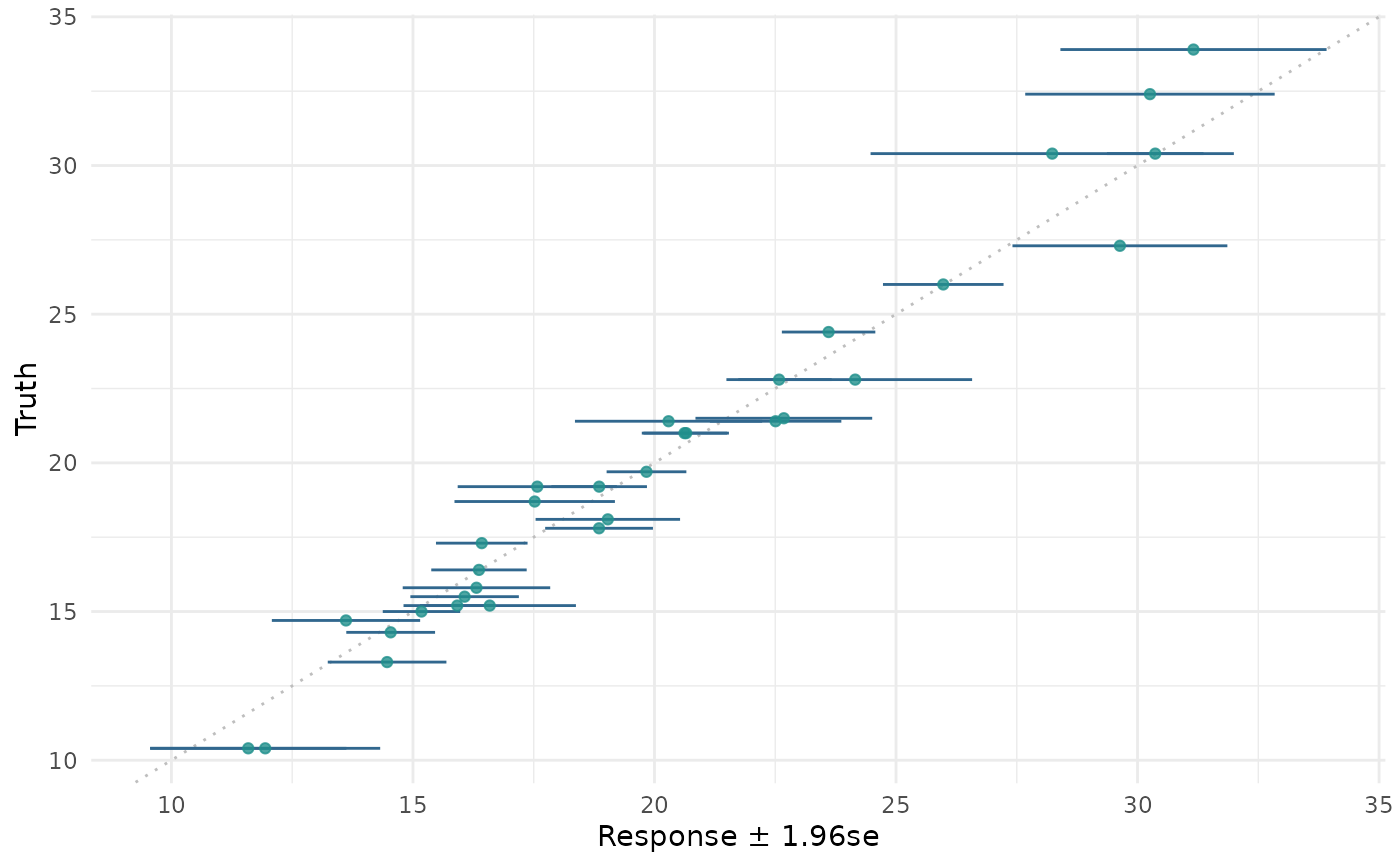Visualizations for mlr3::PredictionRegr. The argument type controls what kind of plot is drawn. Possible choices are:

• "xy" (default): Scatterplot of "true" response vs. "predicted" response. By default a linear model is fitted via geom_smooth(method = "lm") to visualize the trend between x and y (by default colored blue). In addition geom_abline() with slope = 1 is added to the plot. Note that geom_smooth() and geom_abline() may overlap, depending on the given data.

• "histogram": Histogram of residuals: $$r = y - \hat{y}$$.

• "residual": Plot of the residuals, with the response $$\hat{y}$$ on the "x" and the residuals on the "y" axis. By default a linear model is fitted via geom_smooth(method = "lm") to visualize the trend between x and y (by default colored blue).

• "confidence: Scatterplot of "true" response vs. "predicted" response with confidence intervals. Error bars calculated as object$reponse +- quantile * object$se and so only possible with predict_type = "se". geom_abline() with slope = 1 is added to the plot.

## Usage

# S3 method for PredictionRegr
autoplot(
object,
type = "xy",
binwidth = NULL,
theme = theme_minimal(),
quantile = 1.96,
...
)

## Arguments

object
type

(character(1)):
Type of the plot. See description.

binwidth

(integer(1))
Width of the bins for the histogram.

theme

(ggplot2::theme())
The ggplot2::theme_minimal() is applied by default to all plots.

quantile

(numeric(1))
Quantile multiplier for standard errors for type="confidence". Default 1.96.

...

(ignored).

## Value

ggplot2::ggplot().

## Examples

if (requireNamespace("mlr3")) {
library(mlr3)
library(mlr3viz)

learner = lrn("regr.rpart")
object = learner$train(task)$predict(task)

object = learner$train(task)$predict(task)